# 目录

## 参考资料

 为什么要用激活函数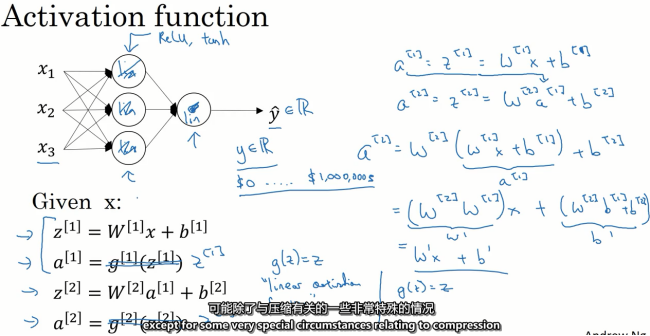sigmod

Sigmod激活函数和导函数分别为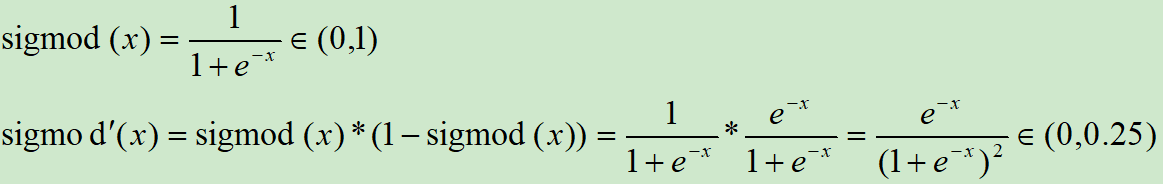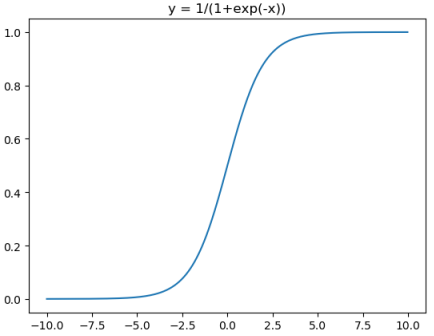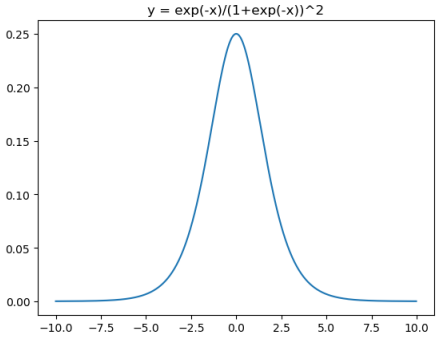from matplotlib import pyplot as plt
import numpy as np
fig = plt.figure()
x = np.arange(-10, 10, 0.025)
plt.plot(x,1/(1+np.exp(-x)))
plt.title("y = 1/(1+exp(-x))")
plt.show()

plt.plot(x,np.exp(-x)/(1+np.exp(-x))**2)
plt.title("y = exp(-x)/(1+exp(-x))^2")
plt.show()
View Code

Sigmod(x)的缺点：

①输出范围在0~1之间，均值为0.5，需要做数据偏移，不方便下一层的学习。

②当x很小或很大时，存在导数很小的情况。另外，神经网络主要的训练方法是BP算法，BP算法的基础是导数的链式法则，也就是多个导数的乘积。而sigmoid的导数最大为0.25，多个小于等于0.25的数值相乘，其运算结果很小。随着神经网络层数的加深，梯度后向传播到浅层网络时，基本无法引起参数的扰动，也就是没有将loss的信息传递到浅层网络，这样网络就无法训练学习了。这就是所谓的梯度消失。

 tanh

tanh是双曲函数中的一个，tanh()为双曲正切。在数学中，双曲正切“tanh”是由双曲正弦和双曲余弦这两种基本双曲函数推导而来。

tanh激活函数和导函数分别为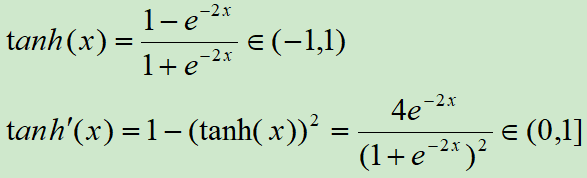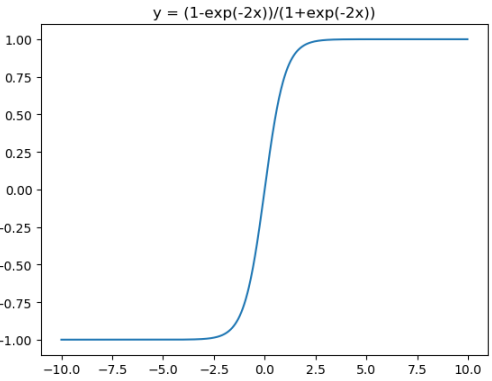from matplotlib import pyplot as plt
import numpy as np
fig = plt.figure()
x = np.arange(-10, 10, 0.025)
plt.plot(x,(1-np.exp(-2*x))/(1+np.exp(-2*x)))
plt.title("y = (1-exp(-2x))/(1+exp(-2x))")
plt.show()

plt.plot(x,4*np.exp(-2*x)/(1+np.exp(-2*x))**2)
plt.title("y = 4exp(-2x)/(1+exp(-2x))^2")
plt.show()
View Code

Sigmod(x)和tanh(x)都有一个缺点：在深层网络的学习中容易出现梯度消失，造成学习无法进行。

 ReLU

ReLU激活函数和导函数分别为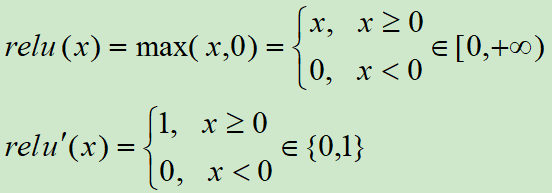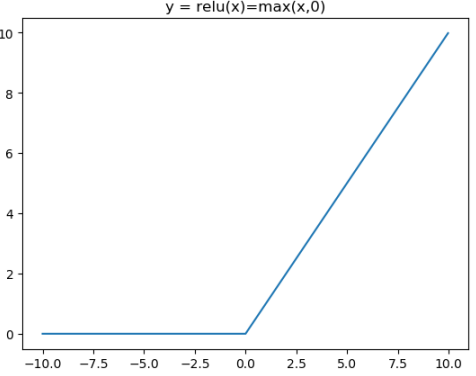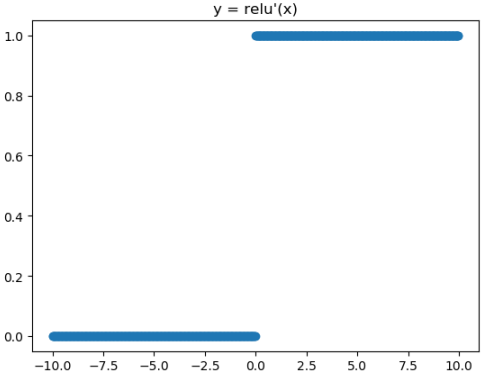from matplotlib import pyplot as plt
import numpy as np
fig = plt.figure()
x = np.arange(-10, 10, 0.025)
plt.plot(x,np.clip(x,0,10e30))
plt.title("y = relu(x)=max(x,0)")
plt.show()

from matplotlib import pyplot as plt
plt.plot(x,x>0,"o")
plt.title("y = relu'(x)")
plt.show()
View Code

Relu的一个缺点是当x为负时导数等于零，但是在实践中没有问题，也可以使用leaky Relu。

 LeakyReLU

Leaky ReLU激活函数和导函数分别为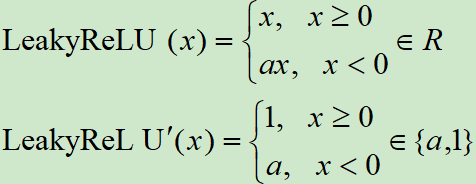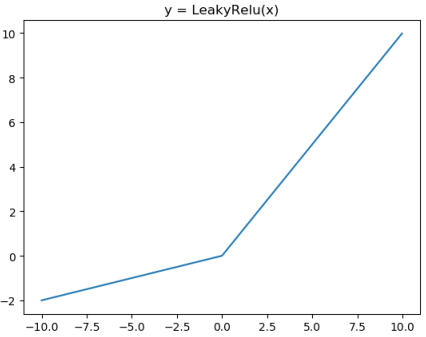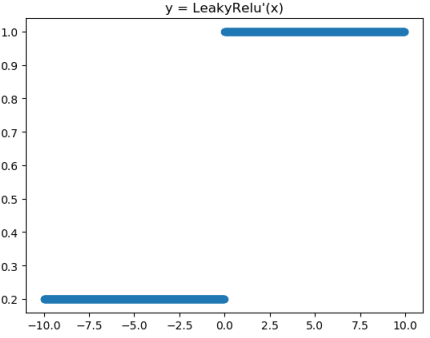from matplotlib import pyplot as plt
import numpy as np
fig = plt.figure()
x = np.arange(-10, 10, 0.025)
a = 0.2
plt.plot(x,x*np.clip((x>=0),a,1))
plt.title("y = LeakyRelu(x)")
plt.show()

from matplotlib import pyplot as plt
plt.plot(x,np.clip((x>=0),a,1),"o")
plt.title("y = LeakyRelu'(x)")
plt.show()
View Code

 ReLU6

Relu在x>0的区域使用x进行线性激活，有可能造成激活后的值太大，影响模型的稳定性，为抵消ReLU激励函数的线性增长部分，可以使用Relu6函数

ReLU激活函数和导函数分别为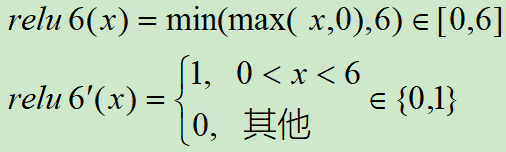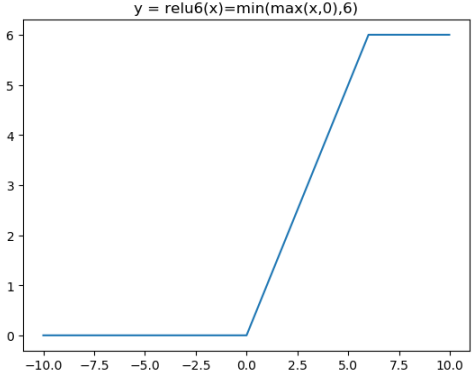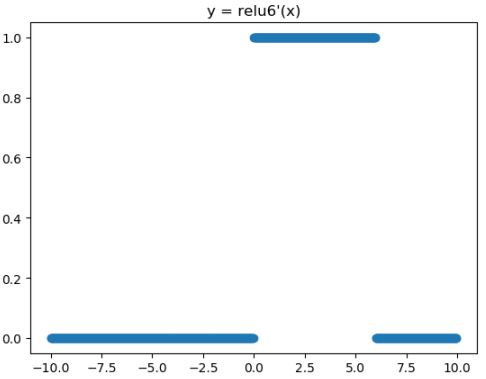from matplotlib import pyplot as plt
import numpy as np
fig = plt.figure()
x = np.arange(-10, 10, 0.025)
plt.plot(x,np.clip(x,0,6))
plt.title("y = relu6(x)=min(max(x,0),6)")
plt.show()

from matplotlib import pyplot as plt
plt.plot(x,(x>0)&(x<6),"o")
plt.title("y = relu6'(x)")
plt.show()
View Code

 参考资料

https://www.cnblogs.com/mengnan/p/9480804.html

《图解深度学习与神经网络：从张量到TensorFlow实现》_张平

posted @ 2019-07-04 14:58  黎明程序员  阅读(16693)  评论(0编辑  收藏  举报